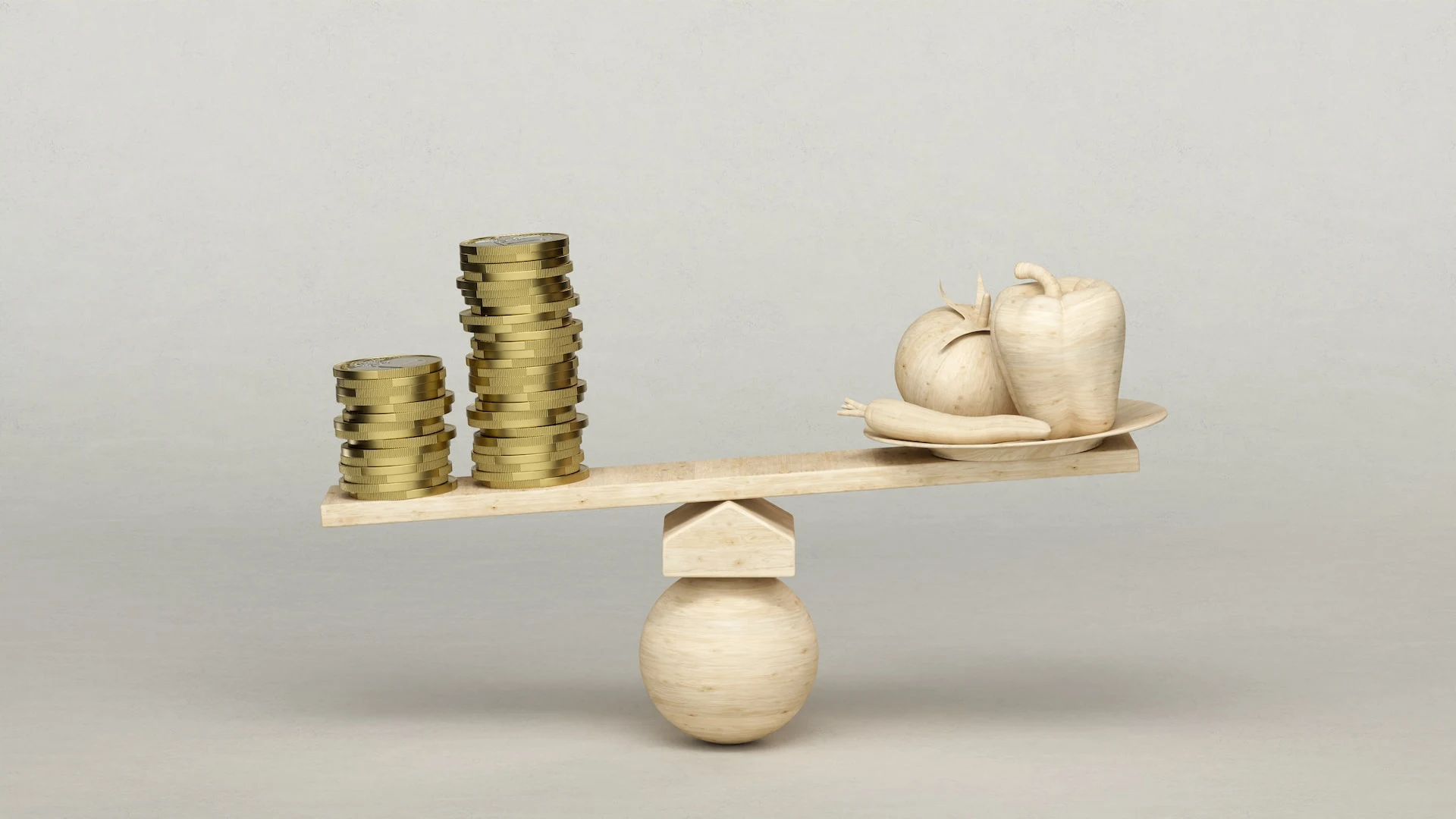Fixed Deposits are a term investment provided by numerous banks and financial institutions. These deposits offer a high-interest rate, subject to specific rules and regulations. You can calculate the exact interest rate with the help of an FD calculator. An FD calculator is an easy-to-use tool that you can use online.Here are steps to calculator the interest rate on FD:

## Understanding Fixed Deposits

Fixed Deposits are a safe and reliable investment tool that helps you grow your savings. The interest rate on your deposit depends entirely on your chosen tenure and its frequency of interest payouts. The FD formula for the interest calculation is:

A = P(1+r/n)^n*t

• A = Maturity amount
• P = Principal amount
• R = Interest rate
• T = No. of years
• N = Compound interest frequency

## What is a Fixed Deposit calculator?

The FD calculator assists customers in calculating the total maturity amount for their account based on the interest rate for a specific period. The calculator is easy to use and helps compare several tenures with different interest rates from various banks, making choosing a fixed amount and term smoother. This also offers you the desired maturity amount for your FD.

## How to use the FD calculator to calculate interest?

Using an FD calculator is simple. Enter the FD amount and tenure to determine the receivable amount at maturity. It helps calculate both cumulative and non-cumulative payouts. For instance, here is how you can calculate your interest on Fixed Deposit:

• Choose customer type: Senior citizen or customer below the age of 60 years
• Choose the investment amount: Rs. 15,000 to Rs. 5 crore
• Choose tenure from 12-60 months
• Choose the frequency payout option: Upon maturity, monthly, quarterly, yearly, and half-yearly

Based on your choices, you can see the FD interest rate applicable to your investment type, the total interest rate during your tenure period, and the maturity amount. It also saves the manual work, and you can decide the investment return instantly.

## Why are FD calculators beneficial?

FD calculators are beneficial if you want to determine the total interest rate and the interest amount you will receive once the maturity period reaches. Using the FD calculator, you can choose the exact amount you will get during FD maturity. An online FD calculator saves a lot of time and gives precise calculations. They automate all the work and provide exact data.

## Conclusion

The information provided gives a fair idea of how you can calculate Fixed Deposit interest rates. So, before investing, use an FD interest calculator to understand better how your investments can grow with time.